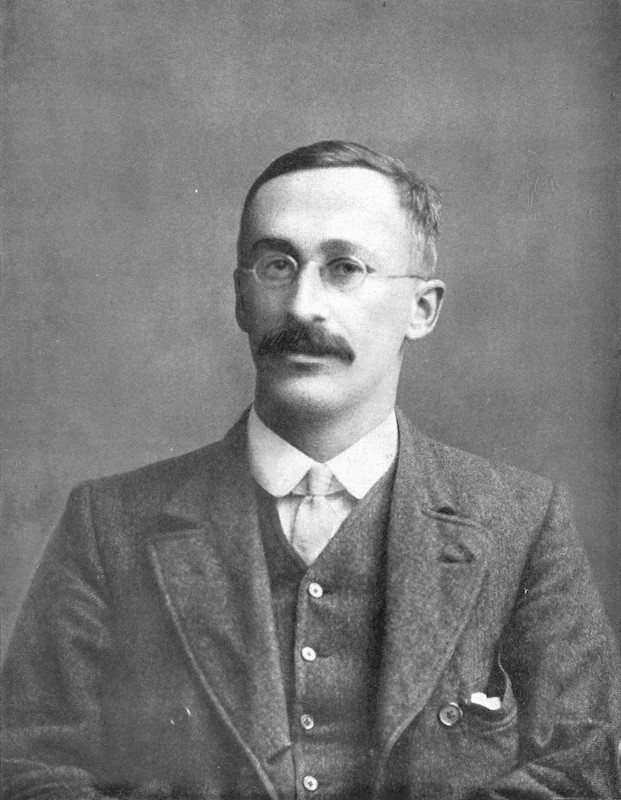# Methodology of Scientific Research

## Hypothesis declaration

We suspect that men and women have different average age

We define what do we want to test, and what is the alternative \begin{aligned} H_0:&μ_f = μ_m\\ H_a:&μ_f ≠ μ_m\\ \end{aligned}

## Experiment

We have two samples (males and females), of size $$N$$ each

We somehow know the variance $$σ^2$$ of each group

We calculate $$Z=(\bar{X}_f - \bar{X}_m)/σ$$ for our samples

This value $$Z$$ follows a Normal distribution (why?)

Under $$H_0$$ the mean of $$Z$$ is 0, but not under $$H_a$$

## Variance of $$Z$$

“Variance of sum is sum of variances” $σ^2=\frac{σ^2_f}{n_f} + \frac{σ^2_m}{n_m}$

Since $$n_f=n_m=N$$ we can write $σ^2=\frac{σ^2_f+σ^2_m}{N}$

## Evaluating the p-value

If $$H_0$$ is true, $$(\bar{X}_f - \bar{X}_m)$$ follows a $$Normal(0, σ^2)$$

Therefore $$Z$$ follows a $$Normal(0, 1)$$

In an experiment we get a fixed value $$z$$

The $$p$$-value is the probability of getting the value $$z$$ or more extreme in our experiment $ℙ(z ≥ \text{abs}(Z)|H_0, σ^2)$

This is a two side test, since $$H_a$$ is $$μ_f ≠ μ_m$$

## In practice

In Excel we use NORMSDIST(z), which is $$ℙ(z < Z |H_0, σ^2)$$

so 1-NORMSDIST(z) will be $$ℙ(z ≥ Z |H_0, σ^2)$$

## Two side test

We must consider both sides

$$ℙ(z ≥ \text{abs}(Z) |H_0, σ)$$ is 2*(1-NORMSDIST(z))

This is the $$p$$-value

# But we do not know $$σ$$

## We do not know the population variance

All we said before is true, but cannot be used directly

Because we do not know the population variance

Thus, we also ignore the population standard deviation

## A solution

The solution is to use the standard deviation of the sample

(do not confuse it with standard deviation of the sample means)

But we have to pay a price: lower confidence

## Sample variance instead of population variance

We need $$ℙ(\text{abs}(z) ≥ \text{abs}(Z)|H_0)$$ but this time we do not know $$σ^2$$

Instead we have $$T=(\bar{X}_f - \bar{X}_m)/S$$, where $S=\sqrt{\frac{\text{stdev}^2(X_f)+\text{stdev}^2(X_m)}{N}}$ and $\text{stdev}^2(X_f)=\frac{1}{N-1}\sum_{x∈ X_f} (x-\bar{x})^2$

## This is not Normal

The value $$T$$ follows a Student’s t-distribution

It is bell shaped, symmetric, but not Normal

## Student’s t-distribution is wider than Normal

Intervals are wider than Normal intervals

(but less than with Chebyshev)

## Who was the Student?He worked at the Guinness Brewery in Ireland

Studied small samples (the chemical properties of barley)

He called it “frequency distribution of standard deviations of samples drawn from a normal population”

Story says that Guinness did not want their competitors to know this quality control, so he used the pseudonym “Student”

## It is a family of Student’s distributions

Here we use the sample standard deviation to approximate the population standard deviation

As we have seen, if the sample is small, these two values may be different

Thus, the Student’s distribution depends on the sample size

More precisely, it depends on the degrees of freedom

## Degrees of Freedom

The key idea is that the sample has $$N$$ elements, but they are constrained by 1 value: the sample average

We say that we have $$N-1$$ degrees of freedom

## Now it is not Normal

For the Normal distribution $ℙ(\text{abs}(z) ≥ \text{abs}(Z) |H_0, σ)$ is calculated as 2*(1-NORMSDIST(z))

For the Student’s t distribution $ℙ(\text{abs}(t) ≥ \text{abs}(T) |H_0)$ is calculated as 2*(1-T.DIST(t))

## Finding Student’s confidence intervals

If we have 95% of population in the center, then we have 2.5% to the left and 2.5% to the right

If the sample standard deviation is $$s$$, the interval is $[\bar{x} - k⋅s,\bar{x} + k⋅s]$

We can find the $$k$$ value if the sample size is 5

T.INV(0.025, 5-1)
T.INV(0.975, 5-1)

## Summary

• Normal distributions are common in nature
• They happen when many things add together
• If the process is Normal, then the sample average is close to the population average
• The confidence interval uses the t-Student distribution